# High School Math : Finding Maxima and Minima

## Example Questions

### Example Question #7 : Pre Calculus

The functionis such that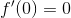When you take the second derivative of the function, you obtain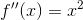What can you conclude about the function at?

The point is an inflection point.

The point is a local minimum.

The point is an absolute maximum.

The point is an absolute minimum.

The point is a local maximum.

The point is an inflection point.

Explanation:

We have a point at which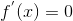. We know from the second derivative test that if the second derivative is negative, the function has a maximum at that point. If the second derivative is positive, the function has a minimum at that point. If the second derivative is zero, the function has an inflection point at that point.

Plug in 0 into the second derivative to obtain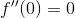So the point is an inflection point.

### Example Question #8 : Pre Calculus

Consider the function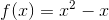Find the maximum of the function on the interval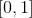.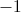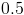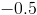Notice that on the interval, the termis always less than or equal to. So the function is largest at the points when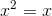. This occurs atand.
Plugging in either 1 or 0 into the original functionyields the correct answer of 0.